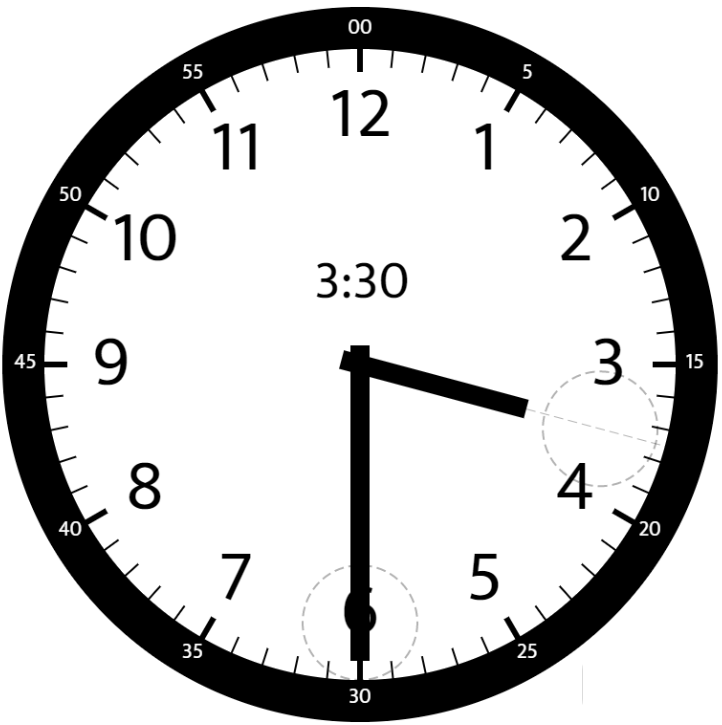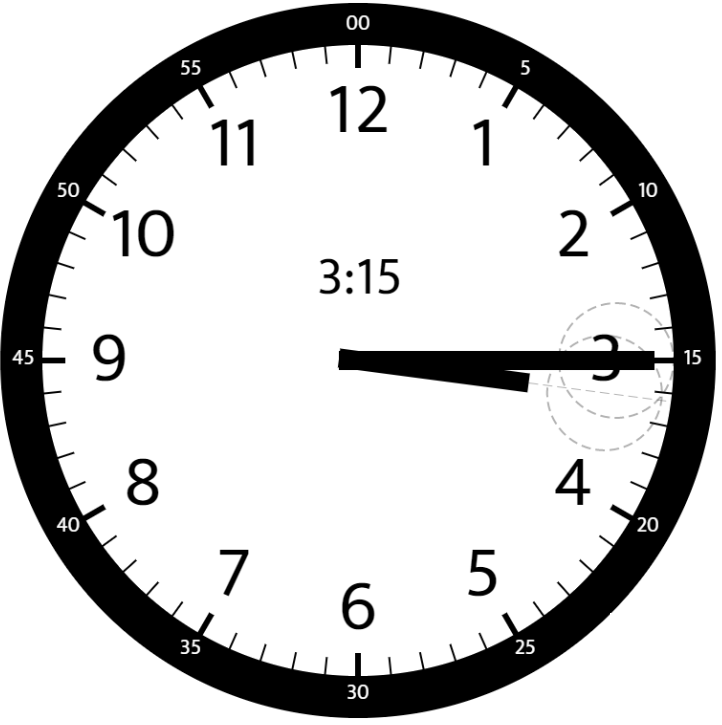1344. Angle Between Hands of a Clock
Medium
1.2K
226

Given two numbers, `hour` and `minutes`, return the smaller angle (in degrees) formed between the `hour` and the `minute` hand.

Answers within `10-5` of the actual value will be accepted as correct.

Example 1:```Input: hour = 12, minutes = 30
Output: 165
```

Example 2:```Input: hour = 3, minutes = 30
Output: 75
```

Example 3:```Input: hour = 3, minutes = 15
Output: 7.5
```

Constraints:

• `1 <= hour <= 12`
• `0 <= minutes <= 59`
Accepted
106.7K
Submissions
168.7K
Acceptance Rate
63.3%

Seen this question in a real interview before?
1/4
Yes
No

Discussion (0)

Related Topics